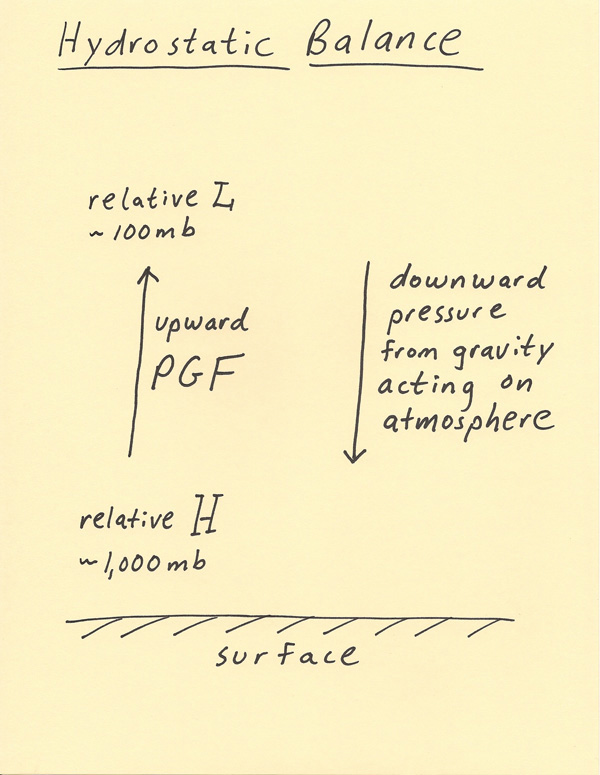[--MAIN HOME--] [--ALL HABYHINTS--] [--FACEBOOK PAGE--]

 HABYTIME MINI LECTURE 28: HYDROSTATIC BALANCE

METEOROLOGIST JEFF HABY

The atmosphere is a thin layer of air that surrounds the Earth’s surface. Although the Earth is zooming around the Sun at over 29 kilometers per second and rotates in a complete circle every 24-hours, the atmosphere stays in place. This is because the atmosphere is moving with the Earth in the same way that a person is moving with an airplane when the airplane is moving 500 miles per hour.

The pressure decreases with height. Thus, there is always lower pressure above higher pressure. Since air moves from high to low pressure, it can be thought that the atmosphere would just drift into outer space to leave the Earth behind. This does not happen because of gravity. Gravity is pushing the atmosphere toward the Earth while the vertical pressure gradient force is pushing the air into space. Gravity and the vertical pressure gradient force are in a balance, thus the atmosphere stays right where it is at.

The diagram below shows these two opposing forces. The vertical pressure gradient force results from pressures always being lower at higher altitudes. The direction of the vertical force is from high pressure toward low pressure (upward from the surface). Gravity is a force that acts on all matter that tries to push matter toward a common center of gravity. The direction of the gravitational force points toward the Earth’s core (downward from the surface) and thus gravity is a force on the air acting to push it toward the ground. These two forces when equally balanced is termed “hydrostatic balance”. Hydrostatic balance results in a stable striation of the atmosphere and no vertical motions. On the whole, vertical motions (upward motion and downward motion) average out to the atmosphere staying in place. Thus, although vertical motions can occur on the mesoscale and synoptic scale, globally these motions average to zero and thus the mass of the atmosphere remains basically constant and the average of upward and downward vertical motions is zero. On a global average, the vertical pressure gradient force and gravity are equal and opposite forces that balance each other which results in the atmosphere staying in place.